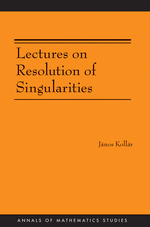Have library access? Log in through your library# Lectures on Resolution of Singularities (AM-166)

János Kollár
Copyright Date: 2007
https://doi.org/10.2307/j.ctt7rptq
Pages: 198
https://www.jstor.org/stable/j.ctt7rptq

## Table of Contents

1. Front Matter
(pp. i-iv)
2. Table of Contents
(pp. v-vi)
3. Introduction
(pp. 1-4)

A complex algebraic varietyXis a subset of complex affinen-space$\mathbb{C}^n$or of complex projectiven-space$\mathbb{C}\mathbb{P}^n$defined by polynomial equations.

A point$x \in X$is called asmoothpoint if, up to a complex analytic local coordinate change,Xlooks like a linear subspace nearx. Otherwise, a point is calledsingular. At singular pointsXmay have self-intersections, it may look like the vertex of a cone, or it can be much more complicated.

Roughly speaking, resolution of singularities asserts that an arbitrary singular varietyXcan be parametrized by a smooth variety X'. That is, all...

4. CHAPTER 1 Resolution for Curves
(pp. 5-66)

Resolution of curve singularities is one of the oldest and prettiest topics of algebraic geometry. In all likelihood, it is also completely explored.

In this chapter I have tried to collect all the different ways of resolving singularities of curves. Each of the thirteen sections contains a method, and some of them contain more than one. These come in different forms: solving algebraic equations by power series, normalizing complex manifolds, projecting space curves, blowing up curves contained in smooth surfaces, birationally transforming plane curves, describing field extensions of Laurent series fields and blowing up or normalizing 1-dimensional rings.

By the...

5. CHAPTER 2 Resolution for Surfaces
(pp. 67-116)

Surface singularities are much more complicated than curves, and resolving them is a rather subtle problem. It seems that surfaces are still special enough to connect resolutions directly to various geometric properties but not general enough to predict which methods generalize to higher dimensions.

One culprit is the existence of a minimal resolution, which is studied in Section 2.2 after some introductory examples in Section 2.1. While minimal resolutions provide a powerful tool for the study of surface singularities, from the point of view of a general resolution method they have been a distraction so far.

Three ways of resolving...

6. CHAPTER 3 Strong Resolution in Characteristic Zero
(pp. 117-196)

The most influential paper on resolution of singularities is Hironaka’s magnum opus [Hir64]. Its starting point is a profound shift in emphasis from resolving singularities of varieties to resolving “singularities of ideal sheaves.” Ideal sheaves of smooth or simple normal crossing divisors are the simplest ones. Locally, in a suitable coordinate system, these ideal sheaves are generated by a single monomial. The aim is to transform an arbitrary ideal sheaf into such a “locally monomial” one by a sequence of blow-ups. Ideal sheaves are much more flexible than varieties, and this opens up new ways of running induction.

Since then,...

7. Bibliography
(pp. 197-202)
8. Index
(pp. 203-208)